Refer to our Texas Go Math Grade 3 Answer Key Pdf to score good marks in the exams. Test yourself by practicing the problems from Texas Go Math Grade 3 Lesson 15.1 Answer Key Classify Quadrilaterals.

Unlock the Problem

An angle is formed by two rays that share an endpoint. Two dimensional figures have angles formed by two line segments that share an endpoint. The shared endpoint is called a vertex. The plural of vertex is vertices.Jason drew this polygon on dot paper.
There are _________ angles.
There are _________ sides.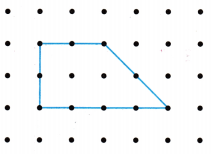There are 4 angles.
There are 4 sides.
Math Idea
A polygon is a closed plane figure with straight sides that are line segments.

Jason’s polygon has 2 right angles. They form square corners. You can use the corner of a sheet of paper to tell if an angle is a right angle. Draw boxes to show the right angles.

Look at Jason s polygon. How many pairs of sides are parallel?Lines that appear to never cross or meet and are always the saine distance apart are parallel lines.
So, Jason’s polygon has ___________ pair of parallel sides.
So, Jason’s polygon has 1 pair of parallel sides.

Some quadrilaterals cannot be classified as a trapezoid, parallelogram, rectangle, square, or rhombus.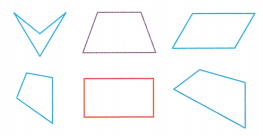__________ sides
__________ angles
4 sides
4 angles

trapezoidexactly _________ pair of opposite sides that are parallel lengths of sides could be the same
exactly 1 pair of opposite sides that are parallel lengths of sides could be the same

parallelogram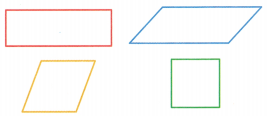__________ pairs of opposite sides that are parallel
__________ pairs of sides that are of equal length.
2 pairs of opposite sides that are parallel
2 pairs of sides that are of equal length.

rectangle
_________ pairs of opposite sides that are parallel
__________ pairs of sides that are of equal length
__________ right angles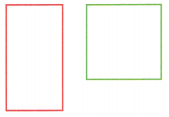2 pairs of opposite sides that are parallel
2 pairs of sides that are of equal length
4 right angles

square
_________ pairs of opposite sides that are parallel
_________ sides that are of equal length
_________ right angles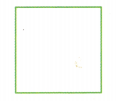2 pairs of opposite sides that are parallel
4 sides that are of equal length
4 right angles

rhombus
_________ pairs of opposite sides that are parallel
_________ sides that are of equal length2 pairs of opposite sides that are parallel
4 sides that are of equal length

Math Talk
Mathematical Processes

Explain why a square can also be named a rectangle or a rhombus.
Explanation:
A square can be described as a rectangle because a square has 4 equal sides
and a rectangle has 2 parallel sides that are equal
In a rhombus 4 sides are equal so, A rhombus can also be called a square
Every Square is a rectangle, but not every rectangle is a square.
A square is a special kind of closed figure with four straight sides and four right angles that also have sides that all have equal length.
Therefore, we can conclude that: A Square is a special kind of rectangle

Share and Show

Look at the quadrilateral at the right.Question 1.
How many right angles are in the quadrilateral? ________ right angles
4 right angles
Explanation:
2 pairs of opposite sides that are parallel
2 pairs of sides that are of equal length
4 right angles

Which sides appear to be parallel? ___________
A and C, B and D are parallel
Explanation:
2 pairs of opposite sides that are parallel
2 pairs of sides that are of equal length
4 right angles

Question 3.
Explanation:
2 pairs of opposite sides that are parallel
2 pairs of sides that are of equal length
4 right angles

Circle all the words that describe the quadrilateral.

Question 4.rectangle
rhombus
square
parallelogram
Explanation:
2 pairs of opposite sides that are parallel
4 sides that are of equal length
4 right angles

Question 5.rhombus
parallelogram
square
rectangle
Explanation:
2 pairs of opposite sides that are parallel
4 sides that are of equal length

Problem Solving

H.O.T. Analyze Write all or some to complete the sentence for 6-9.

Question 6.
The opposite sides of __________ rectangles are parallel.
Explanation:
The opposite sides of all rectangles are parallel.

Question 7.
_________ sides of a rhombus are the same length.
Explanation:
All sides of a rhombus are the same length.

Question 8.
_________ rhombuses are squares.
Explanation:
Some rhombuses are squares.

Question 9.
_________ trapezoids have 1 pair of opposite sides that are parallel.
Explanation:
All trapezoids have 1 pair of opposite sides that are parallel.

Communicate I am a quadrilateral that has no right angles and 4 sides that are of equal length. What figure am I?
Explanation:
2 pairs of opposite sides that are parallel
4 sides that are of equal length

Question 11.
H.O.T. Multi-Step I am a polygon that has 4 sides and 4 angles. All of my angles are right angles. Circle all the figures that I could be.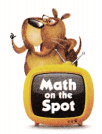rectangle
square
rhombus
trapezoid
parallelogramExplanation:
Circled the figures that I am a polygon that has 4 sides and 4 angles.
All of my angles are right angles.

Fill in the bubble for the correct answer choice.

Question 12.
While playing football after school, Jesse notices that the distance marker contains a quadrilateral with one pair of opposite sides that are parallel. What type of quadrilateral is it?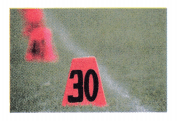(A) rectangle
(B) trapezoid
(C) square
(D) rhombus
Explanation:
Exactly 1 pair of opposite sides that are parallel lengths of sides could be the same

Question 13.
Dean makes a pattern using 10 square tiles. How many pairs of opposite sides are parallel in the 10 square tiles?
(A) 20
(B) 10
(C) 40
(D) 0
Explanation:
Explanation:
2 pairs of opposite sides that are parallel
4 sides that are of equal length
4 right angles

Question 14.
Multi-Step Carina is using scraps of fabric to make a quilt. How many of the fabric scraps are in the shape of rhombuses?(A) 1
(B) 2
(C) 0
(D) 3
Explanation:
Explanation:
2 pairs of opposite sides that are parallel
4 sides that are of equal length

Texas Test Prep

Question 15.(A) There is I right angle.
(B) There are 4 right angles.
(C) There are no right angles.
(D) There are 2 right angles.
Explanation:
The above quadrilateral has 2 right angles
option D is true.

### Texas Go Math Grade 3 Lesson 15.1 Homework and Practice Answer Key

Circle all the words that describe the quadrilateral.

Question 1.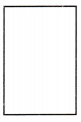parallelogram
trapezoid
rhombus
rectangle
Explanation:
2 pairs of opposite sides that are parallel
2 pairs of sides that are of equal length
4 right angles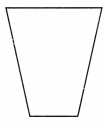parallelogram
trapezoid
rhombus
rectangle
Explanation:
Exactly 1 pair of opposite sides that are parallel lengths of sides could be the same

Problem Solving

Write all or some to complete the sentence for 3-6.

Question 3.
_________ rectangles are squares.
Every Square is a rectangle, but not every rectangle is a square.

Question 4.
__________ angles of a rectangle are right angles.
Explanation:
All angles of a rectangle are right angles.

Question 5.
__________ parallelograms have all 4 sides that are equal in length.
Explanation:
Some parallelograms have all 4 sides that are equal in length.

Question 6.
__________ trapezoids have 2 sides that are equal in length.
Some trapezoids have 2 sides that are equal in length.

Question 7.
I am a polygon that has 2 pairs of opposite sides that are parallel. Circle all of the figures that I could be.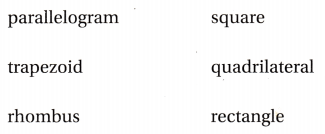Question 8.
I am a quadrilateral that has 4 right angles. My opposite sides are parallel and of equal length. What figure am I?
Explanation:
2 pairs of opposite sides that are parallel
4 sides that are of equal length
4 right angles

Lesson Check

Question 9.
Lily makes an art poster that has exactly one pair of opposite sides that are parallel and 2 sides that are the same length. What figure is Lily’s poster?
(A) trapezoid
(B) rectangle
(C) rhombus
(D) parallelogram
Explanation:
Exactly 1 pair of opposite sides that are parallel lengths of sides could be the same

Desmond draws a polygon that has 4 sides that are the same length and no right angles. How can Desmond classify the polygon he drew?
(A) square
(B) rhombus
(C) rectangle
(D) parallelogram
Explanation:
Explanation:
2 pairs of opposite sides that are parallel
4 sides that are of equal length

Question 11.
Kara makes the kite shown at the right from 4 triangles. What is the shape of Kara’s kite?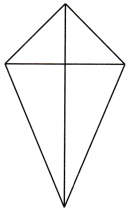(A) square
(B) parallelogram
(C) rhombus
Explanation:
A quadrilateral is a polygon that has exactly four sides. (This also means that a quadrilateral has exactly four vertices, and exactly four angles.)

Question 12.
Multi-Step Ben is making cutouts to use in an art design. How many of the cutouts have the shape of a rectangle?(A) 5
(B) 2
(C) 4
(D) 3